## A Basis for a Vector Space

Let V be a subspace of Rn for some n. A collection B = { v 1, v 2, …, v r } of vectors from V is said to be a basis for V if B is linearly independent and spans V. If either one of these criterial is not satisfied, then the collection is not a basis for V. If a collection of vectors spans V, then it contains enough vectors so that every vector in V can be written as a linear combination of those in the collection. If the collection is linearly independent, then it doesn't contain so many vectors that some become dependent on the others. Intuitively, then, a basis has just the right size: It's big enough to span the space but not so big as to be dependent.

Example 1: The collection {i, j} is a basis for R2, since it spans R 2 and the vectors i and j are linearly independent (because neither is a multiple of the other). This is called the standard basis for R 2. Similarly, the set { i, j, k} is called the standard basis for R 3, and, in general,is the standard basis for R n .

Example 2: The collection { i, i+j, 2 j} is not a basis for R 2. Although it spans R 2, it is not linearly independent. No collection of 3 or more vectors from R 2 can be independent.

Example 3: The collection { i+j, j+k} is not a basis for R 3. Although it is linearly independent, it does not span all of R 3. For example, there exists no linear combination of i + j and j + k that equals i + j + k.

Example 4: The collection { i + j, i − j} is a basis for R 2. First, it is linearly independent, since neither i + j nor i − j is a multiple of the other. Second, it spans all of R 2 because every vector in R 2 can be expressed as a linear combination of i + j and i − j. Specifically, if a i + b j is any vector in R 2, thenif k 1 = ½( a + b) and k 2 = ½( a − b).

A space may have many different bases. For example, both { i, j} and { i + j, i − j} are bases for R 2. In fact, any collection containing exactly two linearly independent vectors from R 2 is a basis for R 2. Similarly, any collection containing exactly three linearly independent vectors from R 3 is a basis for R 3, and so on. Although no nontrivial subspace of R n has a unique basis, there is something that all bases for a given space must have in common.

Let V be a subspace of R n for some n. If V has a basis containing exactly r vectors, then every basis for V contains exactly r vectors. That is, the choice of basis vectors for a given space is not unique, but the number of basis vectors is unique. This fact permits the following notion to be well defined: The number of vectors in a basis for a vector space VR n is called the dimension of V, denoted dim V.

Example 5: Since the standard basis for R 2, { i, j}, contains exactly 2 vectors, every basis for R 2 contains exactly 2 vectors, so dim R 2 = 2. Similarly, since { i, j, k} is a basis for R 3 that contains exactly 3 vectors, every basis for R 3 contains exactly 3 vectors, so dim R 3 = 3. In general, dim R n = n for every natural number n.

Example 6: In R 3, the vectors i and k span a subspace of dimension 2. It is the x−z plane, as shown in Figure .Figure 1

Example 7: The one‐element collection { i + j = (1, 1)} is a basis for the 1‐dimensional subspace V of R 2 consisting of the line y = x. See Figure .Figure 2

Example 8: The trivial subspace, { 0}, of R n is said to have dimension 0. To be consistent with the definition of dimension, then, a basis for { 0} must be a collection containing zero elements; this is the empty set, ø.

The subspaces of R 1, R 2, and R 3, some of which have been illustrated in the preceding examples, can be summarized as follows:Example 9: Find the dimension of the subspace V of R 4 spanned by the vectors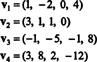The collection { v 1, v 2, v 3, v 4} is not a basis for V—and dim V is not 4—because { v 1, v 2, v 3, v 4} is not linearly independent; see the calculation preceding the example above. Discarding v 3 and v 4 from this collection does not diminish the span of { v 1, v 2, v 3, v 4}, but the resulting collection, { v 1, v 2}, is linearly independent. Thus, { v 1, v 2} is a basis for V, so dim V = 2.

Example 10: Find the dimension of the span of the vectorsSince these vectors are in R 5, their span, S, is a subspace of R 5. It is not, however, a 3‐dimensional subspace of R 5, since the three vectors, w 1, w 2, and w 3 are not linearly independent. In fact, since w 3 = 3w 1 + 2w 2, the vector w 3 can be discarded from the collection without diminishing the span. Since the vectors w 1 and w 2 are independent—neither is a scalar multiple of the other—the collection { w 1, w 2} serves as a basis for S, so its dimension is 2.

The most important attribute of a basis is the ability to write every vector in the space in a unique way in terms of the basis vectors. To see why this is so, let B = { v 1, v 2, …, v r } be a basis for a vector space V. Since a basis must span V, every vector v in V can be written in at least one way as a linear combination of the vectors in B. That is, there exist scalars k 1, k 2, …, k r such that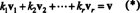To show that no other choice of scalar multiples could give v, assume thatis also a linear combination of the basis vectors that equals v.

Subtracting (*) from (**) yields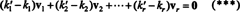This expression is a linear combination of the basis vectors that gives the zero vector. Since the basis vectors must be linearly independent, each of the scalars in (***) must be zero: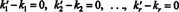Therefore, k′ 1 = k 1, k′ 2 = k 2,…, and k′ r = k r, so the representation in (*) is indeed unique. When v is written as the linear combination (*) of the basis vectors v 1, v 2, …, v r , the uniquely determined scalar coefficients k 1, k 2, …, k r are called the components of v relative to the basis B. The row vector ( k 1, k 2, …, k r ) is called the component vector of v relative to B and is denoted ( v) B . Sometimes, it is convenient to write the component vector as a column vector; in this case, the component vector ( k 1, k 2, …, k r ) T is denoted [ v] B .

Example 11: Consider the collection C = { i, i + j, 2 j} of vectors in R 2. Note that the vector v = 3 i + 4 j can be written as a linear combination of the vectors in C as follows: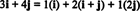and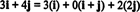The fact that there is more than one way to express the vector v in R 2 as a linear combination of the vectors in C provides another indication that C cannot be a basis for R 2. If C were a basis, the vector v could be written as a linear combination of the vectors in C in one and only one way.

Example 12: Consider the basis B = { i + j, 2 ij} of R 2. Determine the components of the vector v = 2 i − 7 j relative to B.

The components of v relative to B are the scalar coefficients k 1 and k 2 which satisfy the equation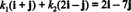This equation is equivalent to the system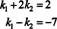The solution to this system is k 1 = −4 and k 2 = 3, so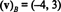Example 13: Relative to the standard basis { i, j, k} = { ê 1, ê 2, ê 3} for R 3, the component vector of any vector v in R 3 is equal to v itself: ( v) B = v. This same result holds for the standard basis { ê 1, ê 2,…, ê n} for every R n .

Orthonormal bases. If B = { v 1, v 2, …, v n } is a basis for a vector space V, then every vector v in V can be written as a linear combination of the basis vectors in one and only one way: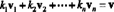Finding the components of v relative to the basis B—the scalar coefficients k 1, k 2, …, k n in the representation above—generally involves solving a system of equations. However, if the basis vectors are orthonormal, that is, mutually orthogonal unit vectors, then the calculation of the components is especially easy. Here's why. Assume that B = {vˆ 1,vˆ 2,…,vˆ n} is an orthonormal basis. Starting with the equation above—with vˆ 1, vˆ 2,…, vˆ n replacing v 1, v 2, …, v n to emphasize that the basis vectors are now assumed to be unit vectors—take the dot product of both sides with vˆ 1:By the linearity of the dot product, the left‐hand side becomes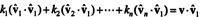Now, by the orthogonality of the basis vectors, vˆ i · vˆ 1 = 0 for i = 2 through n. Furthermore, because vˆ is a unit vector, vˆ 1 · vˆ 1 = ‖vˆ 1‖1 2 = 1 2 = 1. Therefore, the equation above simplifies to the statement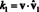In general, if B = { 1, 2,…, n} is an orthonormal basis for a vector space V, then the components, k i , of any vector v relative to B are found from the simple formula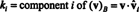Example 14: Consider the vectorsfrom R 3. These vectors are mutually orthogonal, as you may easily verify by checking that v 1 · v 2 = v 1 · v 3 = v 2 · v 3 = 0. Normalize these vectors, thereby obtaining an orthonormal basis for R 3 and then find the components of the vector v = (1, 2, 3) relative to this basis.

A nonzero vector is normalized—made into a unit vector—by dividing it by its length. Therefore,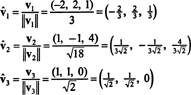Since B = { 1, 2, 3} is an orthonormal basis for R 3, the result stated above guarantees that the components of v relative to B are found by simply taking the following dot products:Therefore, ( v) B = (5/3, 11/(3√2),3/√2), which means that the unique representation of v as a linear combination of the basis vectors reads v = 5/3 1 + 11/(3√2) 2 + 3/√2 3, as you may verify.

Example 15: Prove that a set of mutually orthogonal, nonzero vectors is linearly independent.

Proof. Let { v 1, v 2, …, v r } be a set of nonzero vectors from some R n which are mutually orthogonal, which means that no v i = 0 and v i · v j = 0 for ij. Let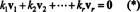be a linear combination of the vectors in this set that gives the zero vector. The goal is to show that k 1 = k 2 = … = k r = 0. To this end, take the dot product of both sides of the equation with v 1: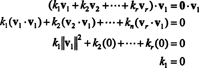The second equation follows from the first by the linearity of the dot product, the third equation follows from the second by the orthogonality of the vectors, and the final equation is a consequence of the fact that ‖ v 12 ≠ 0 (since v 10). It is now easy to see that taking the dot product of both sides of (*) with v i yields k i = 0, establishing that every scalar coefficient in (*) must be zero, thus confirming that the vectors v 1, v 2, …, v r are indeed independent.